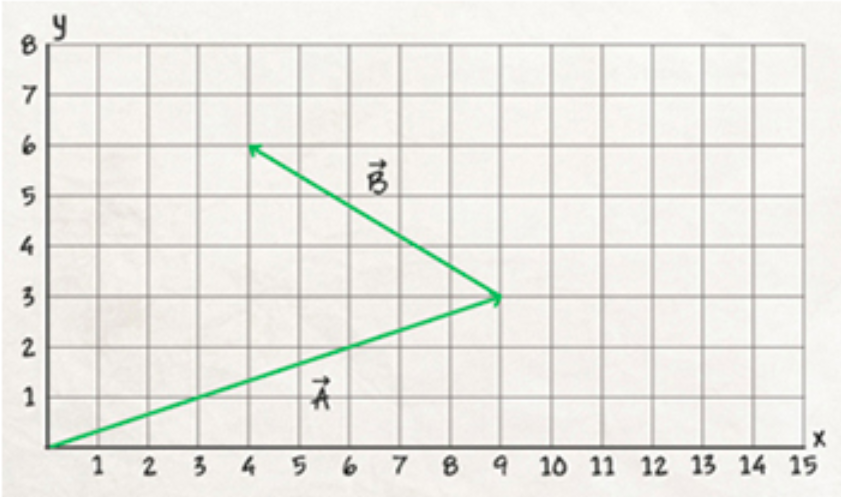# Problem: The sum of the x components of vectors A and B in (Figure 1) is _. Vectors A and B are shown in a tip to tail arrangement. Vector A begins at the origin (0, 0) and ends at the xy coordinate (9, 3). Vector B begins at the tip of vector A and ends at the xy coordinate (4, 6).

###### FREE Expert Solution

When adding vectors graphically, vectors are joined in a head to tail manner.

The tail of the first vector is placed at the origin.

97% (394 ratings)###### Problem Details

The sum of the x components of vectors A and B in (Figure 1) is _. Vectors A and B are shown in a tip to tail arrangement. Vector A begins at the origin (0, 0) and ends at the xy coordinate (9, 3). Vector B begins at the tip of vector A and ends at the xy coordinate (4, 6).